# Thunderstorm

The height of the pole before the storm is 10 m. After a storm when they come to check it they see that on the ground from the pole blows part of the column. Distance from the pole is 3 meters. At how high was the pole broken?

(In fact, a rectangular triangle was created. .. . 10-x, 3 and hypotenuse how much is x).

x =  4.55 m

### Step-by-step explanation:

Try calculation via our triangle calculator.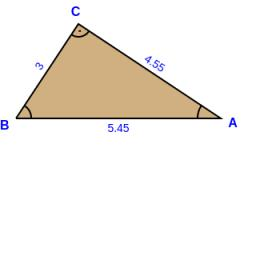Did you find an error or inaccuracy? Feel free to write us. Thank you!Tips to related online calculators
Looking for help with calculating roots of a quadratic equation?
Do you have a linear equation or system of equations and looking for its solution? Or do you have a quadratic equation?
Pythagorean theorem is the base for the right triangle calculator.

#### You need to know the following knowledge to solve this word math problem:

We encourage you to watch this tutorial video on this math problem:

## Related math problems and questions:

• A mast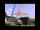A mast 32 meters high was broken by the wind so that its top touches the ground 16 meters from the pole. The still standing part of the mast, the broken part and the ground form a rectangular triangle. At what height was the mast broken?
• Column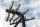Perpendicular pole high 8 m tall broke and its toe fell 2.7 m from the bottom of the pole. At what height above the ground pole broke?
• Bamboo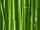Bamboo high 32 feet was at a certain height broken by the wind so the bamboo top reached the ground at a distance of 16 feet from the trunk. At what height from the ground was the bamboo broken?
• Broken treeThe tree was 35 meters high. The tree broke at the height of 10 m above the ground. Top but does not fall off it refuted on the ground. How far from the base of the tree lay its peak?
• Broken treeThe tree is broken at 4 meters above the ground and the top of the tree touches the ground at a distance of 5 from the trunk. Calculate the original height of the tree.
• Free space in the gardenThe grandfather's free space in the garden was in the shape of a rectangular triangle with 5 meters and 12 meters in length. He decided to divide it into two parts and the height of the hypotenuse. For the smaller part creates a rock garden, for the large
• The pole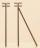The telegraph pole is supported by a 4 m bullet at 3/4 of its height, the end of which is at a distance 2.5 m from the pole post. Calculate the height of the telegraph pole.
• Power line poleFrom point A, the power line pole is seen at an angle of 18 degrees. From point B to which we get when going from point A 30m away from the column at an angle of 10 degrees. Find the height of the power pole.
• There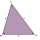There is a stretched steel cable between the 3 columns. The height of the first column is 4 m, the height of the second is 3.5 m. The distance between the first two columns is 2.5 m, the distance between the second and third is 5 m. The heels of all three
• Triangle KLMIn the rectangular triangle KLM, where is hypotenuse m (sketch it!). Find the length of the leg k and the height of triangle h if the hypotenuse's segments are known MK = 5cm and ml = 15 cm.
• Sides of right angled triangleOne leg is 1 m shorter than the hypotenuse, and the second leg is 2 m shorter than the hypotenuse. Find the lengths of all sides of the right-angled triangle.
• Difference of legsIn a right triangle, the length of the hypotenuse is 65 m, and the difference of legs is 23 m. Calculate the perimeter of this triangle.
• Right angled triangleThe hypotenuse of a right triangle is 17 cm long. When we decrease the length of legs by 3 cm, then decrease its hypotenuse by 4 cm. Find the size of its legs.
• ArcCalculate span of the arc, which is part of a circle with diameter d = 20 m and its height is 6 m.
• RT perimeterThe leg of the rectangular triangle is 7 cm shorter than the second leg and 8 cm shorter than the hypotenuse. Calculate the triangle circumference.
• Rectangular base pyramidCalculate an area of the shell of the pyramid with a rectangular base of 2.8 m and 1.4 m and height 2.5 meters.
• Iron pole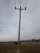The iron pole is in the ground 2/5 of its length, partly above the ground 1/3 is yellow, and the unpainted section is 6 m long. How long is the entire column?